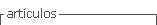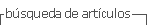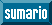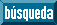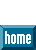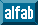## Articulo

•Citado por SciELO
•Accesos

## versión impresa ISSN 0212-7199

#### Resumen

CARRION VALERO, F. et al. Alveolar-arterial gradient of o2 in copd with hypercapnia . An. Med. Interna (Madrid) [online]. 2001, vol.18, n.3, pp.9-14. ISSN 0212-7199.

Objective: This study was conducted to assess whether or not calculation of the alveolar-arterial oxygen tension difference (AaPO2) from arterial blood gas measurements, breathing air, is useful to discriminate patients with hypecapnia associated with obstructive lung disease. Patients and methods: The effect of different values for the respiratory exchange ratio (R) was also analyzed. We calculated the AaPO2 in 45 patients with chronic airway obstruction and hypercapnia (PaCO2 in 45 mmHg) and hemodynamic stability using the standard alveolar gas equation, PAO2 = PIO2 - PACO2 [FIO2 + (1-FIO2)/R];, with R assumed to vary from 0.6 to 1.1 and the simplified alveolar gas equation, PAO2 = PI02 - PACO2/R, with R assumed to be 0.8. It was considered that a patient was correctly classified when the calculated AaPO2 was greater than predicted AaPO2. Results: When the simplified alveolar gas equation was used, 9 (20%) of 45 patients were misclassified, whereas using the standard equation with R assumed to be 0.8, 41 (91%) of 45 patients were correctly classified. Modification of the value of R only allowed improving the classification of two further patients. Conclusions: We conclude that calculation of the AaPO2 using the standard equation and R = 0.8 can be used to classify hypercapnic COPD patients. This laboratory parameter is of sufficient discriminant value to be used for clinical purposes.

Palabras clave : Alveolar-arterial oxygen gradient; Chronic obstructive pulmonary disease (COPD); Hypercapnia.

· resumen en Español     · texto en Español     · Español (pdf )Todo el contenido de esta revista, excepto dónde está identificado, está bajo una Licencia Creative Commons